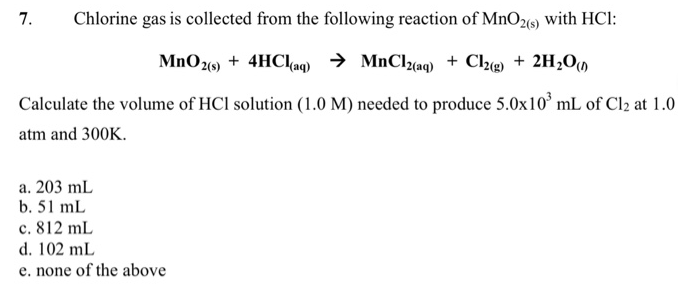Chemistry Practice Problems Gas Stoichiometry Practice Problems Solution: Chlorine gas is collected from the following react...

# Solution: Chlorine gas is collected from the following reaction of MnO2(s) with HCl:MnO2(s) + 4HCl(aq) → MnCl2(aq) + Cl2(g) + 2H2O(l) Calculate the volume of HCl solution (1.0 M) needed to produce 5.0 x 103 mL of Cl2 at 1.0 atm and 300K. a. 203 mL b. 51 mL c. 812 mL d. 102 mL e. none of the above

###### Problem

Chlorine gas is collected from the following reaction of MnO2(s) with HCl:

MnO2(s) + 4HCl(aq) → MnCl2(aq) + Cl2(g) + 2H2O(l)

Calculate the volume of HCl solution (1.0 M) needed to produce 5.0 x 103 mL of Cl2 at 1.0 atm and 300K.

a. 203 mL

b. 51 mL

c. 812 mL

d. 102 mL

e. none of the aboveView Complete Written Solution

Gas Stoichiometry

Gas Stoichiometry

#### Q. Hydrogen and iodine gases react to form hydrogen iodide gas as follows: H2 (g) + I2 (g) → 2HI (g) At a certain temperature and pressure 1.4 L of H 2 r...

Solved • Wed Jul 04 2018 13:50:12 GMT-0400 (EDT)

Gas Stoichiometry

#### Q. What is the total volume of all gases that are produced when 32.0 mL of C3H5(NO3)3 reacts as shown in the reaction below. Assume standard temp and pre...

Solved • Tue Jun 26 2018 15:14:51 GMT-0400 (EDT)

Gas Stoichiometry

#### Q. Chlorine gas reacts with fluorine gas to form chlorine trifluoride. Cl2 (g) + 3F2 (g) → 2 ClF3 (g) A 2.00 L reaction vessel, initially at 298 K, conta...

Solved • Tue Jun 19 2018 15:01:51 GMT-0400 (EDT)

Gas Stoichiometry

#### Q. Ammonium carbonate decomposes upon heating according to the following balanced equation: (NH4)2CO3 (s) → 2 NH3 (g) + CO2 (g) + H2O (g) Calculate the t...

Solved • Tue Jun 19 2018 14:58:19 GMT-0400 (EDT)